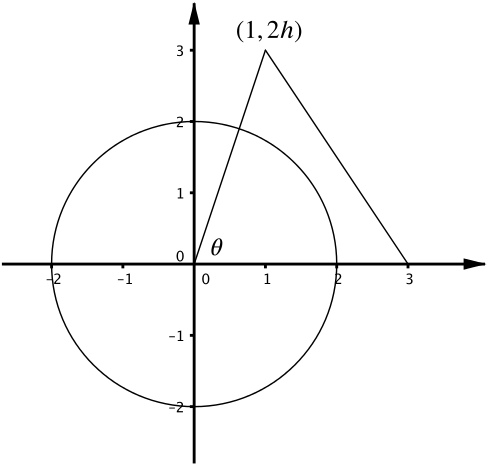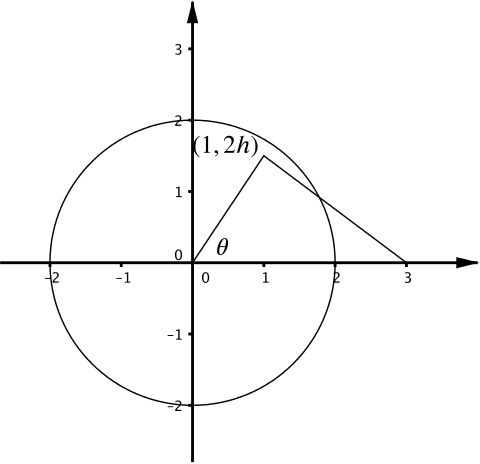Review question

# What's the area inside both the circle $C$ and the triangle $T$? Add to your resource collection Remove from your resource collection Add notes to this resource View your notes for this resource

Ref: R7495

## SolutionDiagram when $h > 2/\sqrt{5}$Diagram when $h < \sqrt{3}/2$

The three corners of a triangle $T$ are $(0,0), (1, 2h), (3,0)$, where $h > 0$. The circle $C$ has equation $x^2 + y^2 = 4$. The angle of the triangle at the origin is denoted as $\theta$. The circle and triangle are drawn in the diagram above for different values of $h$.

1. Express $\tan \theta$ in terms of $h$.

If we construct a triangle at the point $(1,2h)$ we can see that $\tan \theta = 2h$.

1. Show that the point $(1, 2h)$ lies inside $C$ when $h < \sqrt{3}/2$.

The critical point, when $(1,2h)$ lies on the circle, gives us a right-angled triangle with sides $1, 2h$ and hypotenuse $2$.

Using Pythagoras here gives $h = \dfrac{\sqrt{3}}{2}$, and so the point $(1,2h)$ is inside the circle if and only if $h < \dfrac{\sqrt{3}}{2}$.

1. Find the equation of the line connecting $(3,0)$ and $(1,2h)$. Show that this line is tangential to the circle $C$ when $h = 2/\sqrt{5}$.

The equation of the line is $\dfrac{y-0}{x-3}= \dfrac{2h-0}{1-3}$, or $y = -hx+3h$.

Substituting in to $x^2 + y^2 = 4$ gives $x^2 + (-hx+3h)^2 = 4$, and multiplying out gives us $x^2(1+h^2) +x(-6h^2) +(9h^2-4)=0$.

The discriminant here for this quadratic in $x$ is

$36h^4-4(1+h^2)(9h^2-4) = -20h^2+16.$

This is zero if and only if $h = \dfrac{2}{\sqrt{5}}$, and so for this value of $h$, our line is tangential to the circle $C$, since it intersects $C$ in one point only.

Alternatively we can substitute $h = \dfrac{2}{\sqrt{5}}$ into $x^2 + (-hx+3h)^2 = 4$ and observe we get one possible value for $x$.

1. Suppose now that $h > 2/\sqrt{5}$. Find the area of the region inside both $C$ and $T$ in terms of $\theta$.

So the line from (iii) touches the curve. The area inside $C$ and $T$ is then simply a sector of the circle, and so its area is $\dfrac{1}{2}r^2\theta = 2\theta$.

1. Now let $h = 6/7$. Show that the point $(8/5,6/5)$ lies on both the line (from part (iii)) and the circle $C$.

Hence show that the area of the region inside both $C$ and $T$ equals

$\dfrac{27}{35}+2\alpha,$

where $\alpha$ is an angle whose tangent, $\tan \alpha$, you should determine.

You may use the fact that the area of a triangle with corners $(0,0), (a,b), (c,d)$ is $\dfrac{1}{2}\vert ad-bc\vert$.

We have that $\left(\dfrac{8}{5}\right)^2+\left(\dfrac{6}{5}\right)^2=2$, and $\dfrac{6}{5} = -\left(\dfrac{6}{7}\right)\dfrac{8}{5}+3\left(\dfrac{6}{7}\right)$.

Thus when $h = \dfrac{6}{7}, \left(\dfrac{8}{5},\dfrac{6}{5}\right)$ lies on both the line and the circle.

The sector with angle $\alpha$ has area $2\alpha$, where $\tan \alpha = \dfrac{3}{4}$.

The triangular area inside $C$ and $T$ is (using the given formula) $\dfrac{1}{2}\left\vert 1\times \dfrac{6}{5}-\dfrac{12}{7}\times\dfrac{8}{5}\right\vert = \dfrac{27}{35}$.

Thus the area inside $C$ and $T$ is $\dfrac{27}{35}+2\arctan\left(\dfrac{3}{4}\right)$.